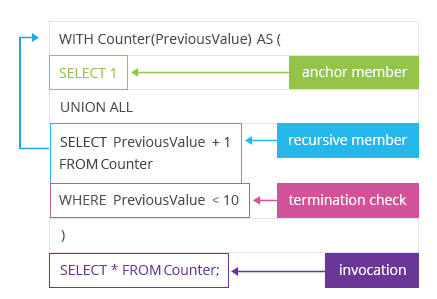End of Summer - hours only!Up to 80% off on all courses and bundles.-Close
Introduction
The very basics
Infinite looping
Processing trees with recursive CTEs
Processing graphs with recursive ctes
Summary

## Instruction

Great! As you could see, we got rows containing the numbers from 1 to 10 without actually using a table. Now, it's time to explain the magic behind that query. Take another look at the template from the previous exercise:A recursive CTE starts just like a normal CTE, but there must be a list of columns inside the parentheses. In this case, our CTE named Counter has only one column, which is the PreviousValue (that is, the value shown in the previous step).

Each correctly constructed recursive CTE has three essential elements inside the parentheses: an anchor member, a recursive member, and a terminator. Let's discuss each of them.

An anchor member is basically something (an anchor point) that we start with. If we want to show the numbers from 1 to 10, we will start with the number 1. You could think of it as the smallest matryoshka doll, which is so small that it has no other matryoshka doll inside. In our case, the anchor member is expressed as

SELECT 1

Then comes the recursive member. The recursive member tells us what to do in each recursive step. In the case of matryoshka dolls, this could be: create a bigger matryoshka doll which will have the previous matryoshka doll inside it. So there we go with numbers: given that you have all the rows with the numbers generated so far, add another row with a number that is bigger by 1 than the number in the most recently generated row. In our case:

SELECT
PreviousValue + 1
FROM Counter


At this point, the CTE Counter refers to itself (FROM Counter).

If we stopped at this point, our query would go on and on, and it would never stop. In our real-life examples, each recursion comes to an end: there is the biggest matryoshka doll that holds all smaller dolls inside itself, just as there is the biggest cone in the Romanesco broccoli.

We need a terminator in recursive CTEs as well: something that will eventually stop our recursion. In this case, it's

WHERE PreviousValue < 10

This means that as long as the previous value is smaller than 10, continue with the recursion; if you reach 10, don't add any new rows. When a recursive CTE has nothing new to add in a given step, it terminates.

## Exercise

Click to continue.